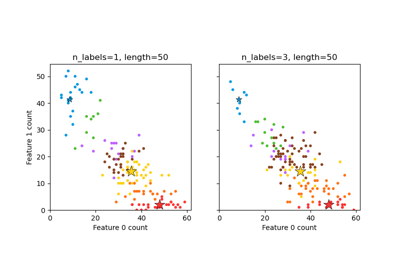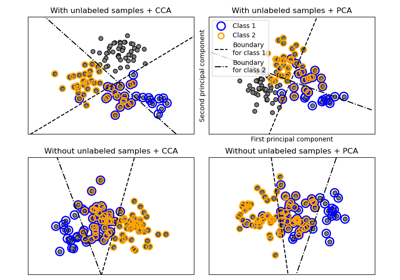# sklearn.datasets.make_multilabel_classification¶

sklearn.datasets.make_multilabel_classification(n_samples=100, n_features=20, *, n_classes=5, n_labels=2, length=50, allow_unlabeled=True, sparse=False, return_indicator='dense', return_distributions=False, random_state=None)[source]

Generate a random multilabel classification problem.

For each sample, the generative process is:
• pick the number of labels: n ~ Poisson(n_labels)

• n times, choose a class c: c ~ Multinomial(theta)

• pick the document length: k ~ Poisson(length)

• k times, choose a word: w ~ Multinomial(theta_c)

In the above process, rejection sampling is used to make sure that n is never zero or more than n_classes, and that the document length is never zero. Likewise, we reject classes which have already been chosen.

Read more in the User Guide.

Parameters
n_samplesint, optional (default=100)

The number of samples.

n_featuresint, optional (default=20)

The total number of features.

n_classesint, optional (default=5)

The number of classes of the classification problem.

n_labelsint, optional (default=2)

The average number of labels per instance. More precisely, the number of labels per sample is drawn from a Poisson distribution with n_labels as its expected value, but samples are bounded (using rejection sampling) by n_classes, and must be nonzero if allow_unlabeled is False.

lengthint, optional (default=50)

The sum of the features (number of words if documents) is drawn from a Poisson distribution with this expected value.

allow_unlabeledbool, optional (default=True)

If True, some instances might not belong to any class.

sparsebool, optional (default=False)

If True, return a sparse feature matrix

New in version 0.17: parameter to allow sparse output.

return_indicator‘dense’ (default) | ‘sparse’ | False

If dense return Y in the dense binary indicator format. If 'sparse' return Y in the sparse binary indicator format. False returns a list of lists of labels.

return_distributionsbool, optional (default=False)

If True, return the prior class probability and conditional probabilities of features given classes, from which the data was drawn.

random_stateint, RandomState instance, default=None

Determines random number generation for dataset creation. Pass an int for reproducible output across multiple function calls. See Glossary.

Returns
Xarray of shape [n_samples, n_features]

The generated samples.

Yarray or sparse CSR matrix of shape [n_samples, n_classes]

The label sets.

p_carray, shape [n_classes]

The probability of each class being drawn. Only returned if return_distributions=True.

p_w_carray, shape [n_features, n_classes]

The probability of each feature being drawn given each class. Only returned if return_distributions=True.

## Examples using sklearn.datasets.make_multilabel_classification¶